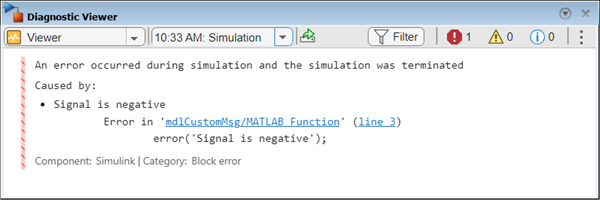Documentation

Customize Diagnostic Messages

The Diagnostic Viewer displays the output of MATLAB® error functions executed during simulation.

You can customize simulation error messages in the following ways by using MATLAB error functions in callbacks, S-functions, or MATLAB Function blocks.

Display Custom Text

This example shows how to can customize the MATLAB function check_signal to display the text Signal is negative.

1. Open the MATLAB Function for editing.2. In the MATLAB Editor, create a function to display custom text.

function y = check_signal(x)
if x < 0
error('Signal is negative');
else
y = x;
end

3. Simulate the model.

A runtime error appears and you are prompted to start the debugger. Click OK.

4. To view the following error in Diagnostic Viewer, close the debugger.Create Hyperlinks to Files, Folders, or Blocks

To create hyperlinks to files, folders, or blocks in an error message, include the path to these items within customized text.

error ('Error reading data from "C:/Work/designData.mat"')Opens designData.data in the MATLAB Editor.
error ('Could not find data in folder "C:/Work"')Opens a Command Window and sets C:\Work as the working folder.
error ('Error evaluating parameter in block "myModel/Mu"')Displays the block Mu in model myModel.

This example shows how to can customize the MATLAB function check_signal to display a hyperlink to the documentation for find_system.

1. Open the MATLAB Function for editing.2. In the MATLAB Editor, create a function to display custom text.

function y = check_signal(x)
if x < 0
error('See help for <a href="matlab:doc find_system">find_system</a>');
else
y = x;
end

3. Simulate the model.

A runtime error appears and you are prompted to start the debugger. Click OK.

4. To view the following error in Diagnostic Viewer, close the debugger.